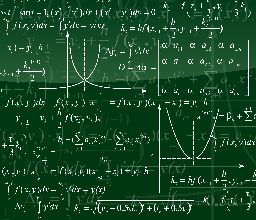# GRE/MAT & Graduate School BlogTo save your time, we've compiled all the essential formulas to know for the GRE, so you could just jump right at it and start your GRE prep.

## 3 Reasons to Memorize GRE Math Formulas

If you're trying to avoid memorizing GRE math formulas, here are 3 reasons why you shouldn't:

• Avoid getting tricked - GRE math formulas are sometimes designed to trick examinees. By understanding the formulas under Quantitative Comparison, Problem Solving, and Data Interpretation, you can place which category the math problem falls in and answer more appropriately.
• Answer math questions faster - There is a time limit on every section, so if you know the math formula, you'd be able to answer the questions quicker and more correctly.
• Get a higher score - Of course, the goal of any test prep is to get a higher score. Understanding math concepts and how each formula works ensure you solve the questions well and in turn, have a better GRE score.

### What Formulas Do I need to Know for the GRE?

The GRE test seems scary because many test-takers haven't faced these math problems for decades.

The GRE Quant section is comprised of math concepts you studied in middle school and high school. These include arithmetic, geometry, algebra, and data analysis (tables, graphs, etc.), among others.

### Is GRE Math Harder than the SAT?

The SAT and ACT encompass higher-level math, but the GRE has advanced passages and questions with challenging vocabulary or tricky wording. This is the reason why many students feel like they were being tricked by the test questions and word problems.

## Most Important GRE Math Formulas and Concepts

If you want to jump-start on your GRE study, this GRE Cheat Sheet should work wonders as a refresher to your geometry and other math knowledge.

### Arithmetic

There is no specific amount of topics covered by a GRE test. As such, make sure to review as many arithmetic concepts as you can find since the Quantitative Comparisons section includes many arithmetic topics such as:

• Types of integers
• Odd and even number properties
• Positives and negatives
• Negative fractions and positive fractions
• Estimation, percentage (percent increase), ratio, rate, and absolute value
• Sequences of numbers

### Geometry

• Learn how to compute the area and perimeter of squares and rectangles, as well as the circumference of circles. For example, to get the perimeter of a rectangle, you need to follow this formula: P=2l+2w. This means you need to multiply the length (l) by 2 and the width (w) by 2, and then add the products together.
• Triangles would have the biggest percent of questions, so refresh on Pythagorean Theorem, Triangle Inequality Theorem, and other triangle concepts (right triangle, isosceles/equilateral triangles).
• Computing the area, e g. length of an arc
• Slope (y=mx + b) and intercept

### Algebra

• The slope of a line, steepness of a line, and other equations of a line
• Linear and quadratic equations (such as x 2 - 3x = 2)
• Simplifying, expanding, and factoring algebraic expressions
• Equations with exponents, fractions, square root, or absolute values
• Graphs of quadratics
• Ratios and proportions
• Finding compound interest

### Data Analysis

The GRE test will always include graphs, tables, charts, and other similar content. You would have to analyze every detail presented, before diving into the question.

This area covers statistics, probability, and interpreting data, so even if the questions seem easy, always take your time to study the data.

### Word Problems

On test day, you'll also face many word problems or problem-solving questions with the basic multiple-choice (5 choices and only one correct answer). Sometimes, you will be asked to enter the answer in a blank or box.

### 10 Must-Know GRE Math Formula

Practice the following formulas as part of your GRE prep. Most of these are included in the Quant section.

1. Average Speed = Total Distance/ Total Time
2. Distance = Rate x Time
3. Probability = Desired outcome/total possible outcome. Learn about:
• Combination: n! /n-k! k!
• Permutation: n! / n-k!
1. Mean/average = sum of terms divided by the number of terms
2. Pythagorean theorem = a2+b2=c2
3. Percentage of a number, percent change (increase or decrease)
4. Compound interest
5. Probability of occasion = {number of ways that fit the requirement}/{number of absolute ways}
6. Laws of square roots = a square =aab=abab=ab
7. Laws of even/odd numbers

Memorizing is just one side of your GRE review. The other side is to use what you learned, and solve as many GRE trial exams as you can.

## Do-it-Yourself GRE Cheat Sheet

Even the best mathematicians need to brush up on a specific math area now and then. The good news is you can DIY the review YOUR way.

The best way to earn a high score number with the GRE is to practice, practice, practice. Make GRE cheat sheet flashcards to help you master the formulas one at a time. Use them in solving free GRE practice tests - you can find these for free virtually everywhere online.

#### About the Author

Leonard Haggin is the founder of Study Prep Lounge, a source of educational information about standardized tests such as the MCAT, LSAT, GRE, SAT, and GMAT.